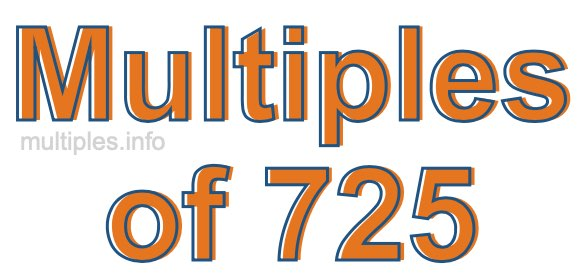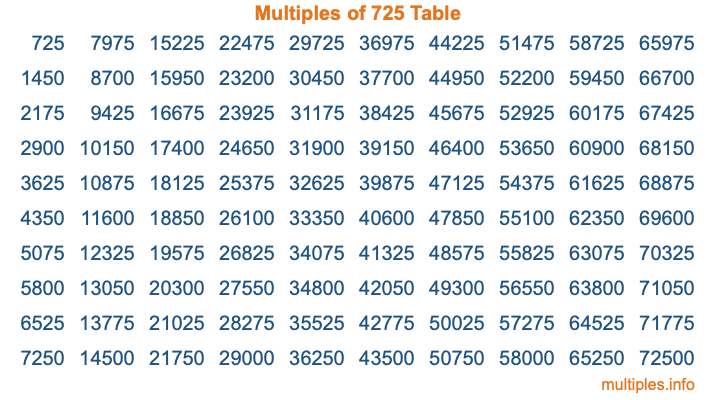Multiples of 725Welcome to the Multiples of 725 page. Here we will first teach you everything you will ever need to know about the multiples of 725, and then give you a study guide summary of everything we taught you to make sure you remember it all. Use this page to look up facts and learn information about the multiples of 725. This page will make you a multiples of seven hundred twenty-five expert!

Definition of Multiples of 725
Multiples of 725 are all the numbers that when divided by 725 equal an integer. Each of the multiples of 725 are called a multiple. A multiple of 725 is created by multiplying 725 by an integer.

Therefore, to create a list of multiples of 725, you start with 1 multiplied by 725, then 2 multiplied by 725, then 3 multiplied by 725, and so on for as long as you want. Thus, the list of the first five multiples of 725 is 725, 1450, 2175, 2900, and 3625. To see a larger list of multiples of 725, see the printable image of Multiples of 725 further down on this page. We also have a category where you can choose any nth multiple of 725.

Multiples of 725 Checker
The Multiples of 725 Checker below checks to see if any number of your choice is a multiple of 725. In other words, it checks to see if there is any number (integer) that when multiplied by 725 will equal your number. To do that, we divide your number by 725. If the the quotient is an integer, then your number is a multiple of 725.

Is  a multiple of 725?

Least Common Multiple of 725 and ...
A Least Common Multiple (LCM) is the lowest multiple that two or more numbers have in common. This is also called the smallest common multiple or lowest common multiple and is useful to know when you are adding our subtracting fractions. Enter one or more numbers below (725 is already entered) to find the LCM.

Check out our LCM Calculator if you need more details about the Least Common Multiple or if you need the LCM for different numbers for adding and subtraction fractions.

nth Multiple of 725
As we stated above, 725 is the first multiple of 725, 1450 is the second multiple of 725, 2175 is the third multiple of 725, and so on. Enter a number below to find the nth multiple of 725.

th multiple of 725

Multiples of 725 vs Factors of 725
725 is a multiple of 725 and a factor of 725, but that is where the similarities end. All postive multiples of 725 are 725 or greater than 725. All positive factors of 725 are 725 or less than 725.

Below is the beginning list of multiples of 725 and the factors of 725 so you can compare:

Multiples of 725: 725, 1450, 2175, 2900, 3625, etc.

Factors of 725: 1, 5, 25, 29, 145, 725

As you can see, the multiples of 725 are all the numbers that you can divide by 725 to get a whole number. The factors of 725, on the other hand, are all the whole numbers that you can multiply by another whole number to get 725.

It's also interesting to note that if a number (x) is a factor of 725, then 725 will also be a multiple of that number (x).

Multiples of 725 vs Divisors of 725
The divisors of 725 are all the integers that 725 can be divided by evenly. Below is a list of the divisors of 725.

Divisors of 725: 1, 5, 25, 29, 145, 725

The interesting thing to note here is that if you take any multiple of 725 and divide it by a divisor of 725, you will see that the quotient is an integer.

Multiples of 725 Table
Below is an image of the first 100 multiples of 725 in a table. The table is in chronological order, column by column. The first column has the first ten multiples of 725, the second column has the next ten multiples of 725, and so on.The Multiples of 725 Table is also referred to as the 725 Times Table or Times Table of 725. You are welcome to print out our table for your studies.

Negative Multiples of 725
Although not often discussed or needed in math, it is worth mentioning that you can make a list of negative multiples of 725 by multiplying 725 by -1, then by -2, then by -3, and so on, to get the following list of negative multiples of 725:

-725, -1450, -2175, -2900, -3625, etc.

Multiples of 725 Summary
Below is a summary of important Multiples of 725 facts that we have discussed on this page. To retain the knowledge on this page, we recommend that you read through the summary and explain to yourself or a study partner why they hold true.

There are an infinite number of multiples of 725.

A multiple of 725 divided by 725 will equal a whole number.

725 divided by a factor of 725 equals a divisor of 725.

The nth multiple of 725 is n times 725.

The largest factor of 725 is equal to the first positive multiple of 725.

725 is a multiple of every factor of 725.

725 is a multiple of 725.

A multiple of 725 divided by a divisor of 725 equals an integer.

725 divided by a divisor of 725 equals a factor of 725.

Any integer times 725 will equal a multiple of 725.

Multiples of a Number
Here you can get the multiples of another number, all with the same attention to detail as we did for multiples of 725 on this page.

Multiples of
Multiples of 726
Did you find our page about multiples of seven hundred twenty-five educational? Do you want more knowledge? Check out the multiples of the next number on our list!